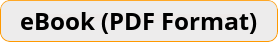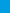# Arithmetic and Pre-Algebra Workbook Comprehensive Activities for Mastering Essential Math SkillsArithmetic and Pre-Algebra Workbook provides students with the confidence and math skills they need to succeed in any math course they choose and prepare them for future study of Algebra, Geometry, Pre-Calculus and Calculus, providing a solid foundation of basic Math topics with abundant exercises for each topic. It is designed to address the needs of math students who must have a working knowledge of basic Math and pre-algebra.\$17.99 \$12.99
-28% only \$17.99 \$12.99
Rated 5.00 out of 5 based on 1 customer rating

Bulk Orders: Need a license for this book?

Arithmetic and Pre-Algebra Workbook provides students with the confidence and math skills they need to succeed in any math course they choose and prepare them for future study of Algebra, Geometry, Pre-Calculus and Calculus, providing a solid foundation of basic Math topics with abundant exercises for each topic. It is designed to address the needs of math students who must have a working knowledge of basic Math and pre-algebra.

Inside the pages of this comprehensive Workbook, students can learn basic math operations in a structured manner with a complete study program to help them understand essential math skills. It also has many exciting features, including:

• Dynamic design and easy-to-follow activities
• A fun, interactive and concrete learning process
• Targeted, skill-building practices
• Fun exercises that build confidence
• All solutions for the exercises are included, so you will always find the answers

## 1 review for Arithmetic and Pre-Algebra Workbook

1.Rated 5 out of 5

I highly recommend this book.

?